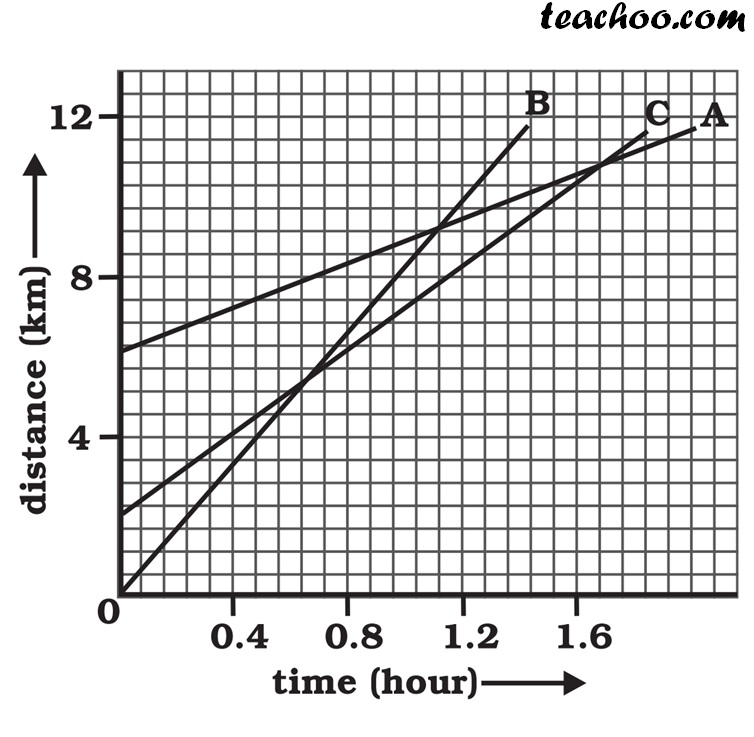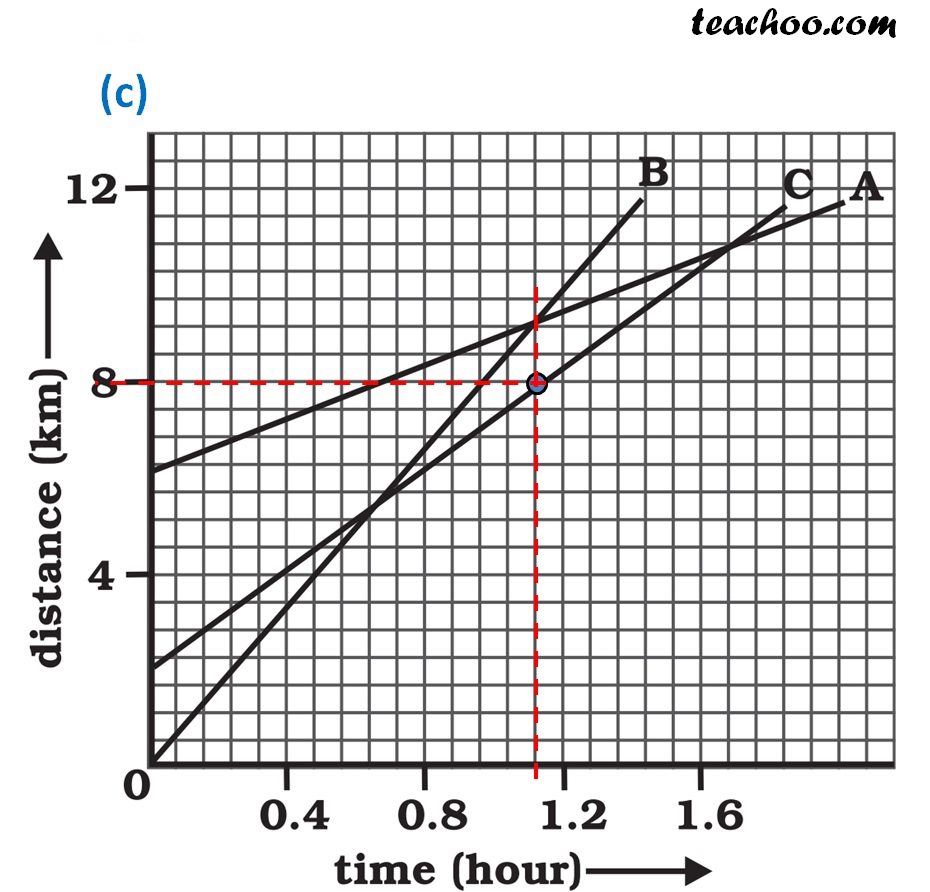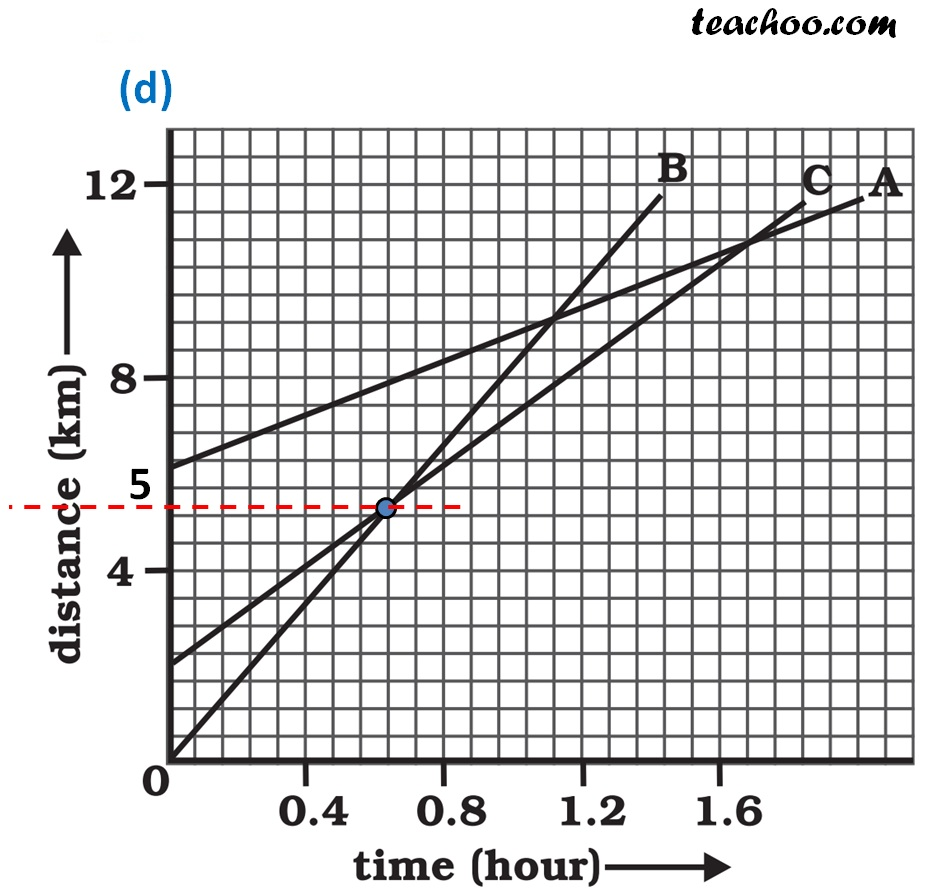NCERT Questions

Class 9
Chapter 8 Class 9 - Motion

## Fig 8.11 shows the distance-time graph of three objects A,B and C. Study the graph and answer the following questions:(a) Which of the three is travelling the fastest?

(b) Are all three ever at the same point on the road?

(c) How far has C travelled when B passes A?

(d) How far has B travelled by the time it passes C?

(a) Speed = Distance/Time

From the graph, we see that

A travels from 6 to 12 km in 2 hours

B travels from 0 to 12 km in 1.4 hours

C travels from 2 to 12 km in 1.6 hours

Since B travels the most distance in the smallest time,

It is travelling the fastest.

(b) All the three objects A,B and C never meet at a single point.

Thus, all the three were never at the same point on the road.When B passes A, we have marked it by the blue dot.

Making a straight line from that point.

Where the straight line intersects line marked by A is the distance travelled.

Thus, distance travelled by A is 8 kmWhen B passes C, we have marked it by the blue dot.

At that point, Distance travelled by B is around 5 km .

Learn in your speed, with individual attention - Teachoo Maths 1-on-1 Class